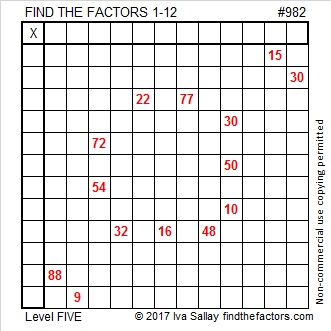# 982 Red-Hot Cinnamon Candy

When I was a child I remember eating a red-hot cinnamon ball around the holidays. I really like cinnamon, but I wasn’t sure I liked how hot the candy was. I hope you enjoy today’s red-hot cinnamon candy puzzle.Print the puzzles or type the solution in this excel file: 12 factors 978-985

Now here’s something interesting about the number 982:

It is palindrome 292 in BASE 20 because 2(20²) + 9(20) + 2(1) = 982.

• 982 is a composite number.
• Prime factorization: 982 = 2 × 491
• The exponents in the prime factorization are 1 and 1. Adding one to each and multiplying we get (1 + 1)(1 + 1) = 2 × 2 = 4. Therefore 982 has exactly 4 factors.
• Factors of 982: 1, 2, 491, 982
• Factor pairs: 982 = 1 × 982 or 2 × 491
• 982 has no square factors that allow its square root to be simplified. √982 ≈ 31.336879